/

### Constrained Quadratic Optimisation: 6. Portfolio optimisation

Next page

6.            Portfolio optimisation

In a (mean-variance) portfolio optimisation context, the objective that we typically want to maximise is the following (or some monotonic equivalent):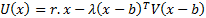Here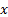are the portfolio weights (so typically we impose at least the following constraint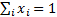),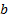is the benchmark (or ‘minimum risk’ portfolio),is a vector of assumed returns on each asset and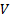is the covariance matrix (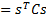, whereis the vector of risks on each asset class, here assumed to be characterised by their volatilities, as this approach is merely a mean-variance one, and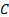their correlation matrix).